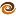# EmptyCrate

#### About Me | Contact Me | Training |ChaiScript.com | YouTube Channel

Standard Algorithms Few C++ developers seem to appreciate that the standard C++ library is actually designed around functional programming principles.

The standard library headers algorithm and functional header files provide functional algorithms and facilitate their use, respectively. As usual we will try to provide a simple example of why we should put to use the functional techniques in the standard library. The most simple case is `for_each` which iterates over the elements in a container and performs some operation on them. Typical example:

``````void printVector(const std::vector<std::string> &data)
{
for (std::vector<std::string>::const_iterator itr = data.begin();
itr != data.end();
++itr)
{
std::cout << *itr << " ";
}
}
``````

A version which uses the `for_each` standard algorithm might look like this:

``````void printElement(const std::string &element)
{
std::cout << element << " ";
}

void printVector(const std::vector<std::string> &data)
{
// For each element in the range [data.begin, data.end) call printElement
for_each(data.begin(), data.end(), &printElement);
}
``````

Why would we choose the second version over the first version? After all, it’s not any shorter. Often times using the standard algorithms does not result in shorter code. There are, however, several advantages to the second version:

### Self Documenting

Using the `for_each` statement makes it clear that you are iterating over a set of iterators and not possibly adding other loop conditions or exiting the loop early in some way.

### Less Error Prone

The single line, easy to read format of the `for_each` statement makes it less likely that you might make a mistake like the following (which assumes that `some_other_data` is also a `std::vector`)

``````for (std::vector<std::string>::const_iterator itr = data.begin();
itr != some_other_data.end();
++itr)
{
}
``````

I’ve personally made that mistake on more than one occasion. It will compile, but will cause an infinite loop.

### More Effecient

The way that standard algorithms are used causes the `.end()` iterator to be cached and not re-looked up for each iteration of the loop.

There are a couple of dozen different standard algorithms covering things such as `find_if` (returns an iterator if an element is found in a range) and `set_difference` (generates the difference between two sorted ranges).

### Boost Utility Libraries

The `boost::bind` and `boost::function` libraries can aid in building functions to pass to the standard algorithms.

### Understanding the Standard Algorithms

Another reason to use the standard algorithms and become familiar with them is to put the techniques of using iterators and functional programming to use in your own code. With these techniques we can write our own generic algorithms. The example above might become:

``````template<typeame InItr>
void printRange(InItr begin, const InItr end)
{
while (begin != end)
{
std::cout << *begin << " ";
++begin; //This is ok because we copy the iterators when we call this function
}
}
``````
My Videos

Related